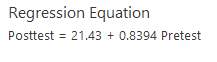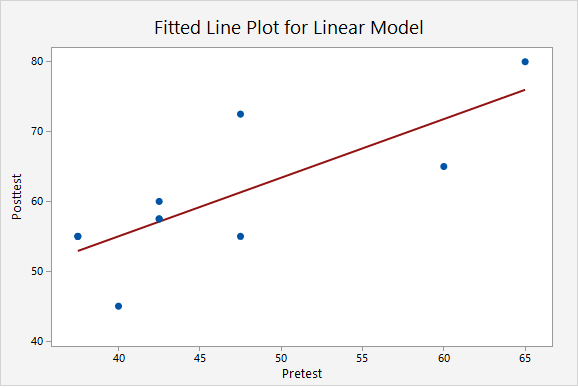# 3.4.3 - Simple Linear Regression

3.4.3 - Simple Linear Regression

Regression uses one or more explanatory variables ($x$) to predict one response variable ($y$). In this course, we will be learning specifically about simple linear regression. The "simple" part is that we will be using only one explanatory variable. If there are two or more explanatory variables, then multiple linear regression is necessary. The "linear" part is that we will be using a straight line to predict the response variable using the explanatory variable. Unlike in correlation, in regression is does matter which variable is called $x$ and which is called $y$. In regression, the explanatory variable is always $x$ and the response variable is always $y$. Both $x$ and $y$ must be quantitative variables.

You may recall from an algebra class that the formula for a straight line is $y=mx+b$, where $m$ is the slope and $b$ is the y-intercept. The slope is a measure of how steep the line is; in algebra, this is sometimes described as "change in y over change in x," ($\frac{\Delta y}{\Delta x}$), or "rise over run." A positive slope indicates a line moving from the bottom left to top right. A negative slope indicates a line moving from the top left to bottom right. The y-intercept is the location on the y-axis where the line passes through. In other words, when $x=0$ then $y=y - intercept$.

In statistics, we use similar formulas:

Simple Linear Regression Line: Sample
$\widehat{y}=a+bx$

$\widehat{y}$ = predicted value of $y$ for a given value of $x$
$a$ = $y$-intercept
$b$ = slope

In a population, the y-intercept is denoted as $\beta_0$ ("beta sub 0") or $\alpha$ ("alpha"). The slope is denoted as $\beta_1$ ("beta sub 1") or just $\beta$ ("beta").

Simple Linear Regression Line: Population
$\widehat{y}=\alpha+\beta x$

Simple linear regression uses data from a sample to construct the line of best fit. But what makes a line “best fit”? The most common method of constructing a simple linear regression line, and the only method that we will be using in this course, is the least squares method. The least squares method finds the values of the y-intercept and slope that make the sum of the squared residuals (also know as the sum of squared errors or SSE) as small as possible.

Residual
The difference between an observed y value and the predicted y value. In other words, $y- \widehat y$. On a scatterplot, this is the vertical distance between the line of best fit and the observation. In a sample this may be denoted as $e$ or $\widehat \epsilon$ ("epsilon-hat") and in a population this may be denoted as $\epsilon$ ("epsilon")
Residual
$e=y-\widehat{y}$

$y$ = actual value of $y$
$\widehat{y}$ = predicted value of $y$

## Example

The plot below shows the line $\widehat{y}=6.5+1.8x$Identify and interpret the y-intercept.

The y-intercept is 6.5. When $x=0$ the predicted value of y is 6.5.

Identify and interpret the slope.

The slope is 1.8. For every one unit increase in x, the predicted value of y increases by 1.8.

Compute and interpret the residual for the point (-0.2, 5.1).

The observed x value is -0.2 and the observed y value is 5.1.

The formula for the residual is $e=y-\widehat{y}$

We can compute $\widehat{y}$ using the regression equation that we have and $x=-0.2$

$\widehat{y}=6.5+1.8(-0.2)=6.14$

Given an x value of -0.2, we would predict this observation to have a y value of 6.14. In reality, they had a y value of 5.1. The residual is the difference between these two values.

$e=y-\widehat{y}=5.1-6.14=-1.04$

The residual for this observation is -1.04. This observation's y value is 1.04 less than predicted given their x value.

## Cautions

• Avoid extrapolation. This means that a regression line should not be used to make a prediction about someone from a population different from the one that the sample used to define the model was from.
• Make a scatterplot of your data before running a regression model to confirm that a linear relationship is reasonable. Simple linear regression constructs a straight line. If the relationship between x and y is not linear, then a linear model is not the most appropriate.
• Outliers can heavily influence a regression model. Recall the plots that we looked at when learning about correlation. The addition of one outlier can greatly change the line of best fit. In addition to examining a scatterplot for linearity, you should also be looking for outliers.

Later in the course, we will devote a week to correlation and regression.

# 3.4.3.1 - SLR in Minitab Express

3.4.3.1 - SLR in Minitab Express

## MinitabExpress – Simple Linear Regression

We previously created a scatterplot of quiz averages and final exam scores and observed a linear relationship. Here, we will use quiz scores to predict final exam scores.

1. Open the data set:
2. On a PC or Mac: Select STATISTICS > Regression > Simple Regression
3. Double click Final in the box on the left to insert it into the Response (Y) box on the right
4. Double click Quiz_Average in the box on the left to insert it into the Predictor (X) box on the right
5. Under the Graphs tab, click the box for Residual plots (We'll learn more about these in Lesson 10)
6. Click OK

This should result in the following output:

Analysis of Variance
Regression 1 2663.66 2663.66 28.24 <0.0001
Error 48 4527.06 94.31
Total 49 7190.72
Model Summary
9.71152 37.04% 35.73%
Coefficients
Term Coef SE Coef T-Value P-Value
Constant 12.12 11.94 1.01 0.3153
Quiz_Average 0.7513 0.1414 5.31 <0.0001
Regression Equation

Final = 12.12 + 0.7513 Quiz_Average

Fits and Diagnostics for Unusual Observations
Obs Final Fit Resid Std Resid
11 49 70.4975 -21.4975 -2.25 R
40 80 61.2158 18.7842 2.03 R
47 37 59.5050 -22.5050 -2.46 R

R Large residual

Video Walkthrough

Select your operating system below to see a step-by-step guide for this example.

In the output in the above example we are given a simple linear regression model of Final = 12.12 + 0.7513 Quiz_Average

This means that the y-intercept is 12.12 and the slope is 0.7513.

# 3.4.3.2 - Example: Interpreting Output

3.4.3.2 - Example: Interpreting Output

This example uses the "CAOSExam" dataset available from http://www.lock5stat.com/datapage.html.

CAOS stands for Comprehensive Assessment of Outcomes in a First Statistics course. It is a measure of students' statistical reasoning skills. Here we have data from 10 students who took the CAOS at the beginning (pre-test) and end (post-test) of a statistics course.

Research question: How can we use students' pre-test scores to predict their post-test scores?

Minitab Express was used to construct a simple linear regression model. The two pieces of output that we are going to interpret here are the regression equation and the scatterplot containing the regression line.Let's work through a few common questions.

What is the regression model?

The "regression model" refers to the regression equation. This is $\widehat {posttest}=21.43 + 0.8394(Pretest)$

Identify and interpret the slope.

The slope is 0.8694. For every one point increase in a student's pre-test score, their predicted post-test score increases by 0.8694 points.

Identify and interpret the y-intercept.

The y-intercept is 21.43. A student with a pre-test score of 0 would have a predicted post-test score of 21.43.  However, in this scenario, we should not actually use this model to predict the post-test score of someone who scored 0 on the pre-test because that would be extrapolation. This model should only be used to predict the post-test score of students from a comparable population whose pre-test scores were between approximately 35 and 65.

One student scored 60 on the pre-test and 65 on the post-test. Calculate and interpret that student's residual.

This student's observed x value was 60 and their observed y value was 65.

$e=y- \widehat y$

We have y.  We can compute $\widehat y$ using the x value and regression equation that we have.

$\widehat y = 21.43 + 0.8394(60) = 71.794$

$e=65-71.794=-6.794$

This student's residual is -6.794. They scored 6.794 points lower on the post-test than we predicted given their pre-test score.

  Link ↥ Has Tooltip/Popover Toggleable Visibility